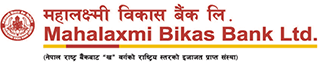# EMI Calculator

The value entered must be a number that is greater than or equal to 0 and less than or equal to 50000000.
%
p.a.
The value entered must be a number that is greater than or equal to 1 and less than or equal to 10.
Years
The value between 1 to 30 Years.
• Principal Rs.10,00,000
• Interest Payable Rs. 0
• Tax Amount Rs. 0
• Total Amount Rs. 0
The value entered must be a number that is greater than or equal to 0 and less than or equal to 50000000.
%
p.a.
The value entered must be a number that is greater than or equal to 1 and less than or equal to 20.
Years
The value between 1 to 30 Years.
• Monthly EMI Rs. 0
• Principal Rs. 0
• Interest Payable Rs. 0
• Total Amount Payable Rs. 0Quick Access